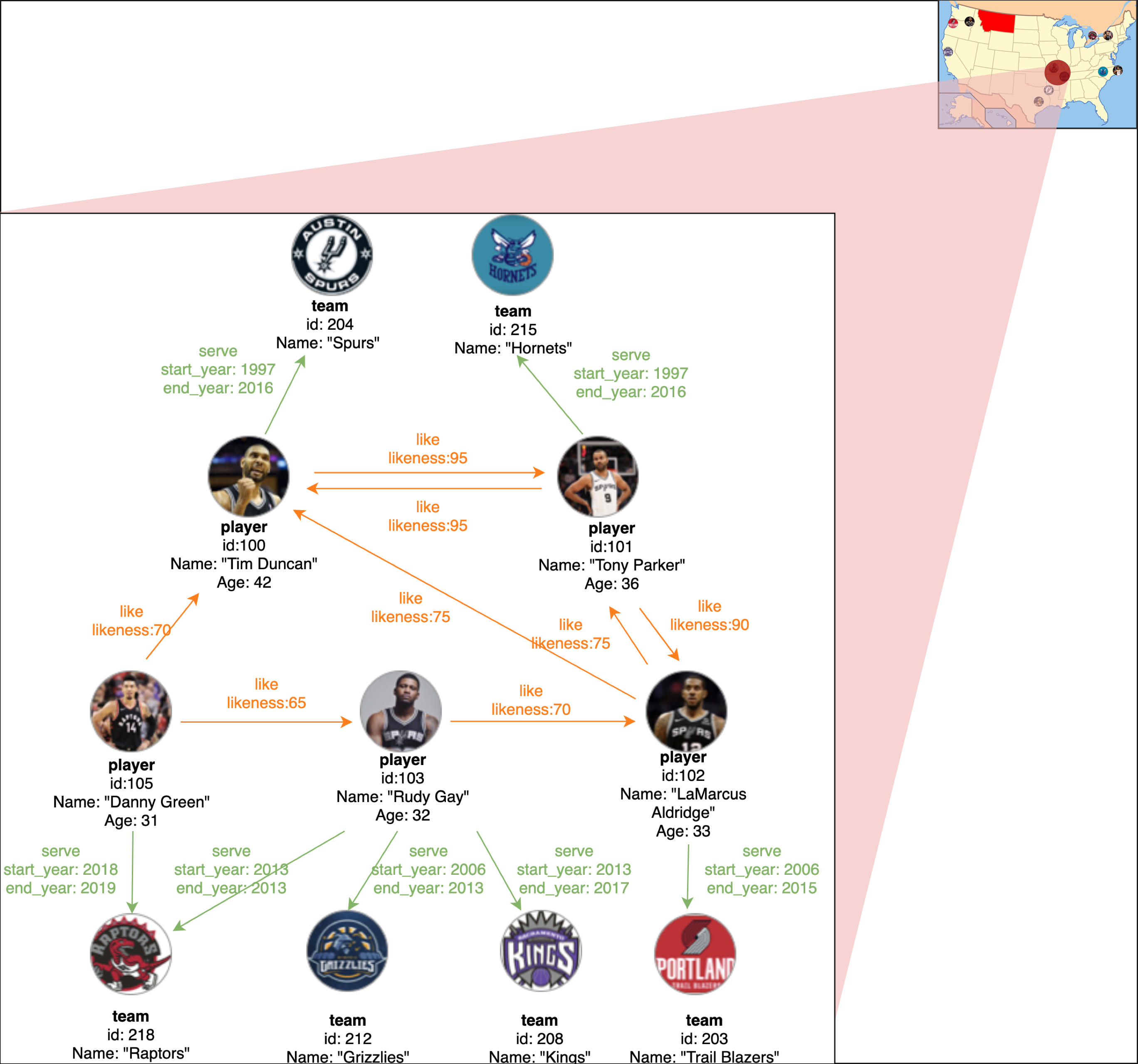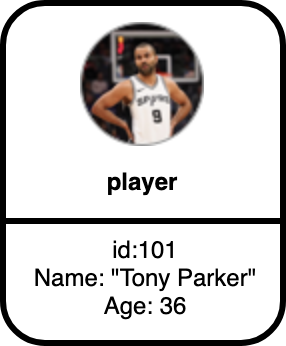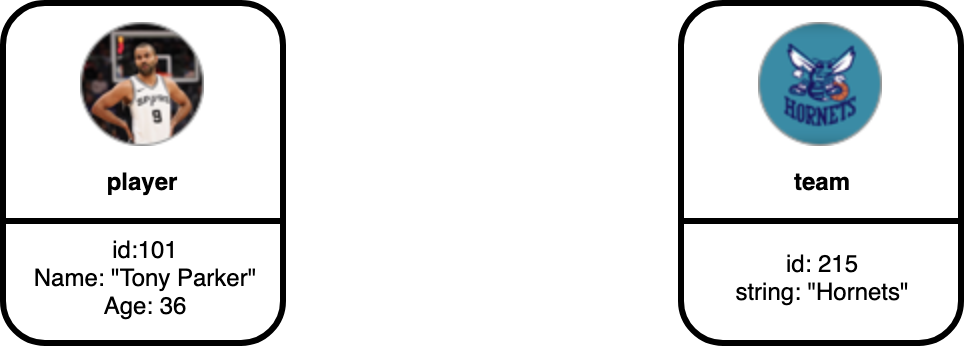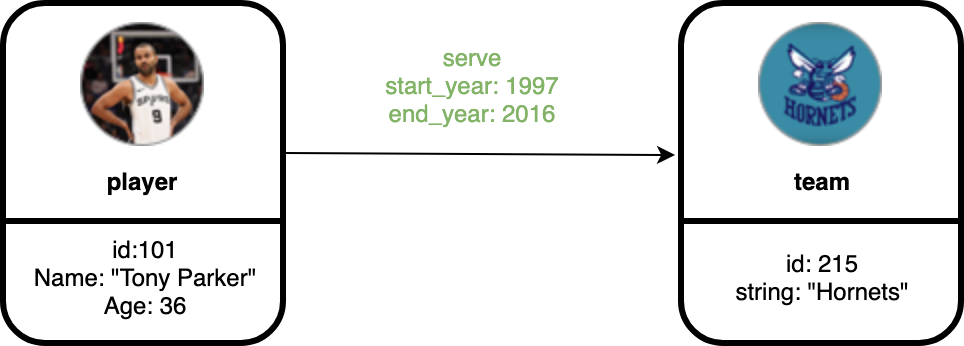# Graph Data Modeling¶

This guide is designed to walk you through the graph data modeling of Nebula Graph. Basic concepts of designing a graph data model will be introduced.

## Graph Space¶

Graph Space is a physically isolated space for different graph. It is similar to database in MySQL.

## Directed Property Graph¶

The data model handled by the Nebula Graph is a directed property graph, whose edges are directional and there could be properties on both edges and vertices. It can be represented as: G = < V, E, PV, PE >, where V is a set of nodes aka vertices, E is a set of directional edges, PV represents properties on vertices, and PE is the properties on edges.

We will use the example graph below to introduce the basic concepts of property graph:In the preceding picture, we have a data set about the players and teams information of NBA. We can see the eleven vertices are classified to two kinds, i.e. player and name while the fifteen edges are classified to serve and like.

To better understand the elements of a graph data model, let us walk through each concept of the example graph.

## Vertices¶

Vertices are typically used to represent entities in the real world. In Nebula Graph, vertices are identified with vertex identifiers (i.e. VIDs). The `VID` must be unique in the graph space. In the preceding example, the graph contains eleven vertices.## Tags¶

In Nebula Graph, vertex properties are clustered by tags. One vertex can have one or more tags. In the preceding example, the vertices have tags player and team.## Edge¶

Edges are used to connect vertices. Each edge usually represents a relationship or a behavior between two vertices. In the preceding example, edges are serve and like.## Edge Type¶

`Each edge` is an instance of an edge type. Our example uses serve and like as edge types. Take edge serve for example, in the preceding picture, vertex `101` (represents a player) is the source vertex and vertex `215` (represents a team) is the target vertex. We see that vertex `101` has an outgoing edge while vertex `215` has an incoming edge.

## Properties of Vertices and Edges¶

Both vertices and edges can have properties. Properties are described with key value pairs. In our example graph, we have used the properties `id`, `name` and `age` on player, `id` and `name` on team, and `likeness` on like edge.

## Edge Rank¶

Edge rank is an immutable user-assigned 64-bit signed integer. It affects the edge order of the same edge type between two vertices. The edge with a higher rank value comes first. When not specified, the default rank value is zero. The current sorting basis is "binary coding order", i.e. 0, 1, 2, ... 9223372036854775807, -9223372036854775808, -9223372036854775807, ..., -1. In addition to an edge type, the edge between two vertices must have an edge rank. The edge rank is a 64-bit integer assigned by the user; if not specified, the edge rank defaults to 0.

An edge can be represented uniquely with the [source vertex, edge type, edge rank, destination vertex].

The edge rank affects the edge order of the same edge type between two vertices. The edge with a higher rank value comes first.

The current sorting basis is "binary coding order", i.e. 0, 1, 2, ... 9223372036854775807, -9223372036854775808, -9223372036854775807, ..., -1.

## Schema¶

In Nebula Graph, schema refers to the definition of properties (name, type, etc.). Like `MySQL`, Nebula Graph is a strong typed database. The name and data type of the properties should be determined before the data is written.